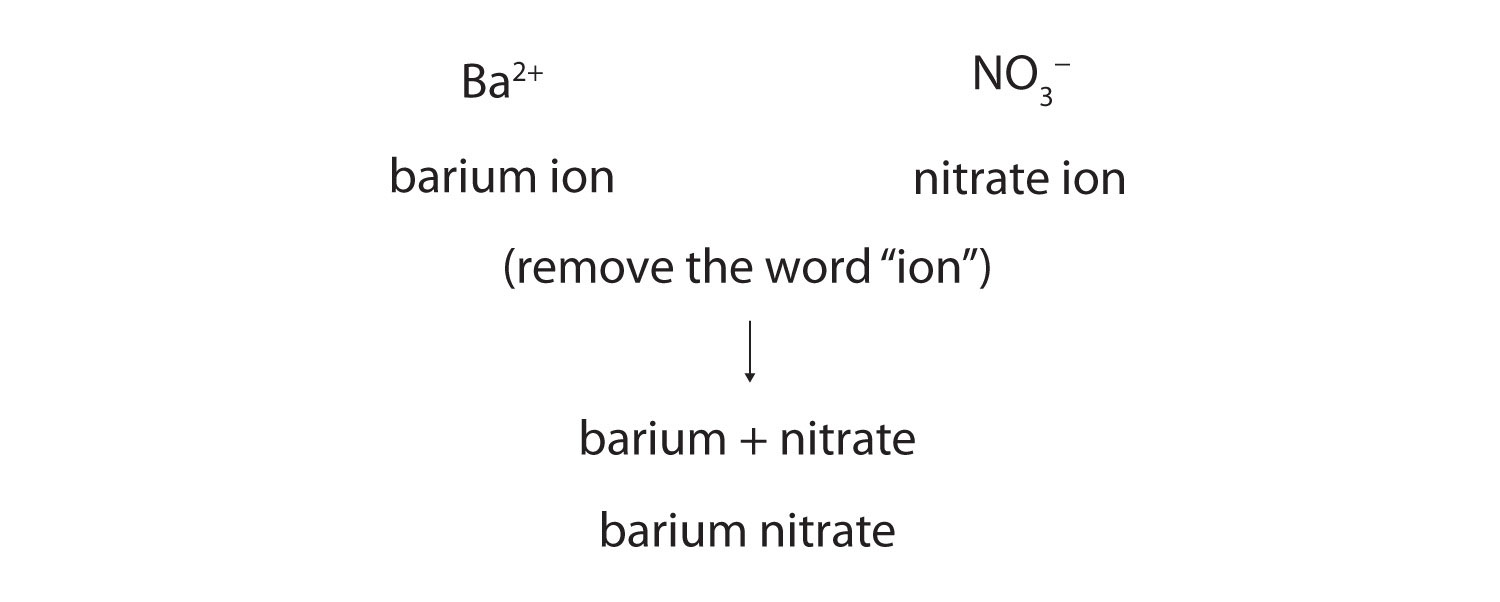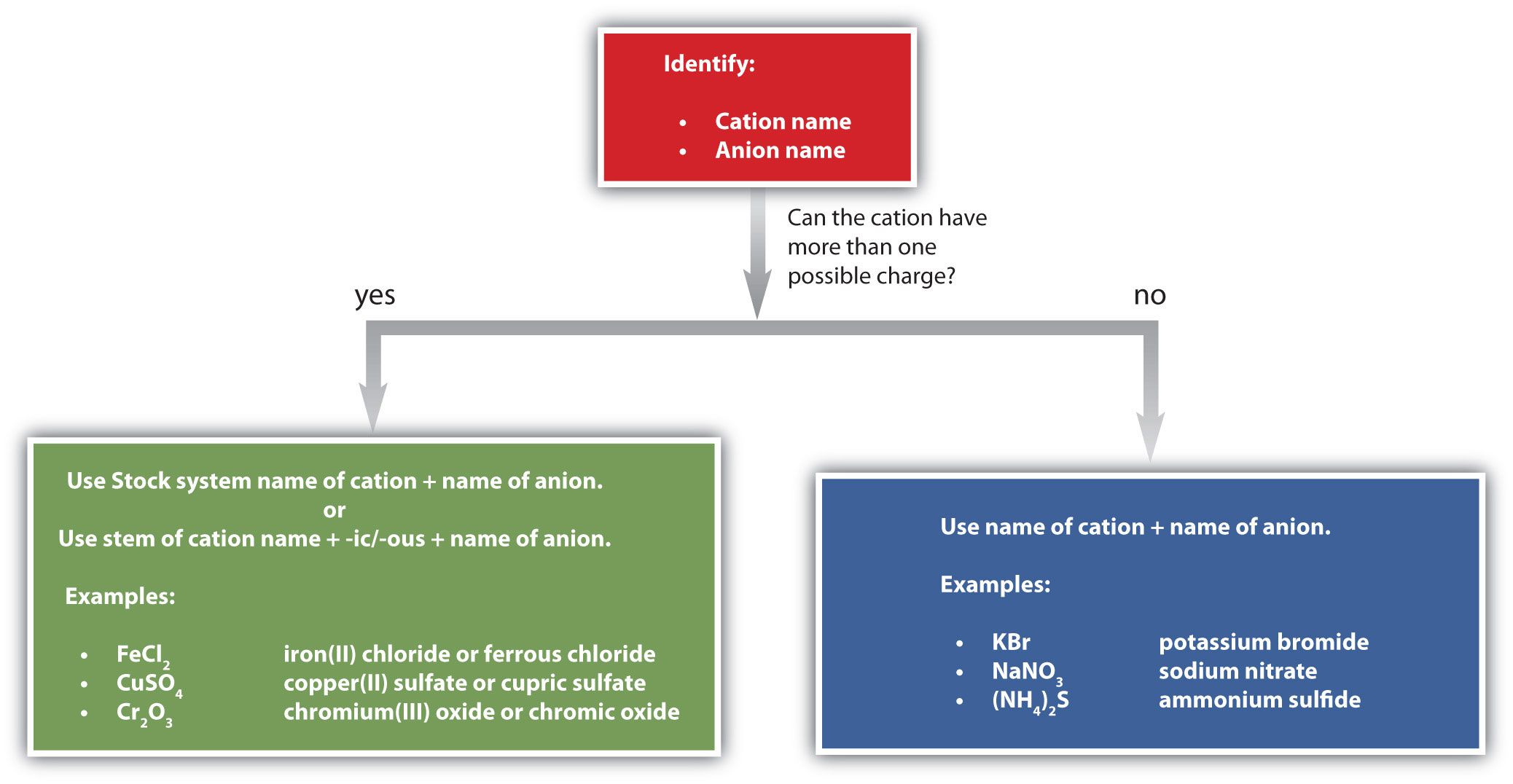## 3.4 Ionic Nomenclature

### Learning Objective

1. Use the rules for naming ionic compounds.

After learning a few more details about the names of individual ions, you will be a step away from knowing how to name ionic compounds. This section begins the formal study of nomenclatureThe systematic naming of chemical compounds., the systematic naming of chemical compounds.

## Naming Ions

The name of a monatomic cation is simply the name of the element followed by the word ion. Thus, Na+ is the sodium ion, Al3+ is the aluminum ion, Ca2+ is the calcium ion, and so forth.

We have seen that some elements lose different numbers of electrons, producing ions of different charges (Figure 3.3 "Predicting Ionic Charges"). Iron, for example, can form two cations, each of which, when combined with the same anion, makes a different compound with unique physical and chemical properties. Thus, we need a different name for each iron ion to distinguish Fe2+ from Fe3+. The same issue arises for other ions with more than one possible charge.

There are two ways to make this distinction. In the simpler, more modern approach, called the Stock systemThe system of indicating a cation’s charge with roman numerals., an ion’s positive charge is indicated by a roman numeral in parentheses after the element name, followed by the word ion. Thus, Fe2+ is called the iron(II) ion, while Fe3+ is called the iron(III) ion. This system is used only for elements that form more than one common positive ion. We do not call the Na+ ion the sodium(I) ion because (I) is unnecessary. Sodium forms only a 1+ ion, so there is no ambiguity about the name sodium ion.

The second system, called the common system, is not conventional but is still prevalent and used in the health sciences. This system recognizes that many metals have two common cations. The common system uses two suffixes (-ic and -ous) that are appended to the stem of the element name. The -ic suffix represents the greater of the two cation charges, and the -ous suffix represents the lower one. In many cases, the stem of the element name comes from the Latin name of the element. Table 3.2 "The Common System of Cation Names" lists the elements that use the common system, along with their respective cation names.

Table 3.2 The Common System of Cation Names

Element Stem Charge Name
iron ferr- 2+ ferrous ion
3+ ferric ion
copper cupr- 1+ cuprous ion
2+ cupric ion
tin stann- 2+ stannous ion
4+ stannic ion
4+ plumbic ion
chromium chrom- 2+ chromous ion
3+ chromic ion
gold aur- 1+ aurous ion
3+ auric ion

The name of a monatomic anion consists of the stem of the element name, the suffix -ide, and then the word ion. Thus, as we have already seen, Cl is “chlor-” + “-ide ion,” or the chloride ion. Similarly, O2− is the oxide ion, Se2− is the selenide ion, and so forth. Table 3.3 "Some Monatomic Anions" lists the names of some common monatomic ions.

Table 3.3 Some Monatomic Anions

Ion Name
F fluoride ion
Cl chloride ion
Br bromide ion
I iodide ion
O2− oxide ion
S2− sulfide ion
P3− phosphide ion
N3− nitride ion

The polyatomic ions have their own characteristic names, as we saw in Table 3.1 "Some Polyatomic Ions".

### Example 6

Name each ion.

1. Ca2+
2. S2−
3. SO32−
4. NH4+
5. Cu+

Solution

1. the calcium ion
2. the sulfide ion (from Table 3.3 "Some Monatomic Anions")
3. the sulfite ion (from Table 3.1 "Some Polyatomic Ions")
4. the ammonium ion (from Table 3.1 "Some Polyatomic Ions")
5. the copper(I) ion or the cuprous ion (Figure 3.5 "Charges of the Monatomic Ions" shows that copper can form cations with either a 1+ or 2+ charge, so we have to specify which charge this ion has)

Name each ion.

1. Fe2+

2. Fe3+

3. SO42−

4. Ba2+

5. HCO3

### Example 7

Write the formula for each ion.

1. the bromide ion
2. the phosphate ion
3. the cupric ion
4. the magnesium ion

Solution

1. Br
2. PO43−
3. Cu2+
4. Mg2+

### Skill-Building Exercise

Write the formula for each ion.

1. the fluoride ion

2. the carbonate ion

3. the stannous ion

4. the potassium ion

## Naming Compounds

Now that we know how to name ions, we are ready to name ionic compounds. We do so by placing the name of the cation first, followed by the name of the anion, and dropping the word ion from both parts.

For example, what is the name of the compound whose formula is Ba(NO3)2?The compound’s name does not indicate that there are two nitrate ions for every barium ion. You must determine the relative numbers of ions by balancing the positive and negative charges.

If you are given a formula for an ionic compound whose cation can have more than one possible charge, you must first determine the charge on the cation before identifying its correct name. For example, consider FeCl2 and FeCl3. In the first compound, the iron ion has a 2+ charge because there are two Cl ions in the formula (1− charge on each chloride ion). In the second compound, the iron ion has a 3+ charge, as indicated by the three Cl ions in the formula. These are two different compounds that need two different names. By the Stock system, the names are iron(II) chloride and iron(III) chloride. If we were to use the stems and suffixes of the common system, the names would be ferrous chloride and ferric chloride, respectively.

### Example 8

Name each ionic compound, using both Stock and common systems if necessary.

1. Ca3(PO4)2
2. (NH4)2Cr2O7
3. KCl
4. CuCl
5. SnF2

Solution

1. calcium phosphate
2. ammonium dichromate (the prefix di- is part of the name of the anion, as in Table 3.1 "Some Polyatomic Ions")
3. potassium chloride
4. copper(I) chloride or cuprous chloride
5. tin(II) fluoride or stannous fluoride

### Skill-Building Exercise

Name each ionic compound, using both Stock and common systems if necessary.

1. ZnBr2

2. Fe(NO3)3

3. Al2O3

4. AuF3

5. AgF

Figure 3.7 "A Guide to Naming Simple Ionic Compounds" is a synopsis of how to name simple ionic compounds.

Figure 3.7 A Guide to Naming Simple Ionic CompoundsFollow these steps to name a simple ionic compound.

### Concept Review Exercises

1. Briefly describe the process for naming an ionic compound.

2. In what order do the names of ions appear in the names of ionic compounds?

3. Which ionic compounds can be named using two different systems? Give an example.

1. Name the cation and then the anion but don’t use numerical prefixes.

2. the cation name followed by the anion name

3. Ionic compounds in which the cation can have more than one possible charge have two naming systems. FeCl3 is either iron(III) chloride or ferric chloride (answers will vary).

### Key Takeaway

• Each ionic compound has its own unique name that comes from the names of the ions.

### Exercises

1. Name each ion.

1. Ra2+
2. P3−
3. H2PO4
4. Sn4+
2. Name each ion.

1. Cs+
2. As3−
3. HSO4
4. Sn2+
3. Name the ionic compound formed by each pair of ions.

1. Na+ and Br
2. Mg2+ and Br
3. Mg2+ and S2−
4. Name the ionic compound formed by each pair of ions.

1. K+ and Cl
2. Mg2+ and Cl
3. Mg2+ and Se2−
5. Name the ionic compound formed by each pair of ions.

1. Na+ and N3−
2. Mg2+ and N3−
3. Al3+ and S2−
6. Name the ionic compound formed by each pair of ions.

1. Li+ and N3−
2. Mg2+ and P3−
3. Li+ and P3−
7. Name the ionic compound formed by each pair of ions. Use both the Stock and common systems, where appropriate.

1. Fe3+ and Br
2. Fe2+ and Br
3. Au3+ and S2−
4. Au+ and S2−
8. Name the ionic compound formed by each pair of ions. Use both the Stock and common systems, where appropriate.

1. Cr3+ and O2−
2. Cr2+ and O2−
3. Pb2+ and Cl
4. Pb4+ and Cl
9. Name the ionic compound formed by each pair of ions. Use both the Stock and common systems, where appropriate.

1. Cr3+ and NO3
2. Fe2+ and PO43−
3. Ca2+ and CrO42−
4. Al3+ and OH
10. Name the ionic compound formed by each pair of ions. Use both the Stock and common systems, where appropriate.

1. NH4+ and NO3
2. H+ and Cr2O72−
3. Cu+ and CO32−
4. Na+ and HCO3
11. Give two names for each compound.

1. Al(HSO4)3
2. Mg(HSO4)2
12. Give two names for each compound.

1. Co(HCO3)2
2. LiHCO3

2. the phosphide ion
3. the dihydrogen phosphate ion
4. the tin(IV) ion or the stannic ion
1. sodium bromide
2. magnesium bromide
3. magnesium sulfide
1. sodium nitride
2. magnesium nitride
3. aluminum sulfide
1. iron(III) bromide or ferric bromide
2. iron(II) bromide or ferrous bromide
3. gold(III) sulfide or auric sulfide
4. gold(I) sulfide or aurous sulfide
1. chromium(III) nitrate or chromic nitrate
2. iron(II) phosphate or ferrous phosphate
3. calcium chromate
4. aluminum hydroxide
1. aluminum hydrogen sulfate or aluminum bisulfate
2. magnesium hydrogen sulfate or magnesium bisulfate